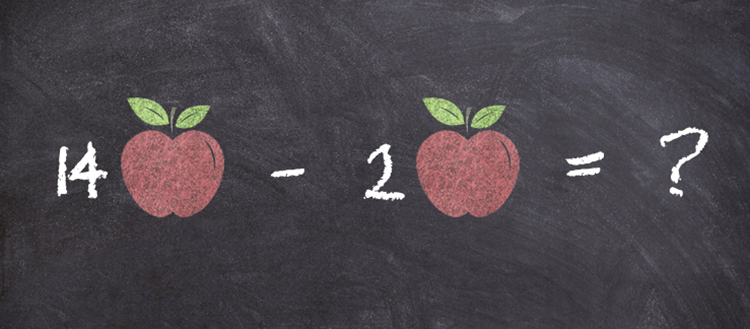# Expert mathematicians stumped by simple subtractions

### UNIGE researchers have shown that our general knowledge about the world interferes with our ability to solve basic mathematical problems, even among experts in the field.Mathematical thought is seen as the pinnacle of abstract thinking. But are we capable of filtering out our knowledge about the world to prevent it from interfering with our calculations? Researchers from the University of Geneva (UNIGE), Switzerland, and the University of Bourgogne Franche-Comté, France, have demonstrated that our ability to solve mathematical problems is influenced by non-mathematical knowledge, which often results in mistakes. The findings, published in Psychonomic Bulletin & Review, indicate that high-level mathematicians can be duped by some aspects of their knowledge about the world and fail to solve primary school-level subtraction problems. It follows that this bias needs to be factored into the way mathematics is taught.

Maths teaching at school usually draws on examples taken from everyday life. Whether it’s adding up oranges and apples to make a pie or dividing a bunch of tulips by the number of vases for a floral arrangement, we master mathematics with the help of concrete examples. But to what extent do the examples chosen affect a child’s ability to use the mathematical concepts in new contexts?

Researchers from UNIGE and the University of Bourgogne Franche-Comté tested the degree to which our worldly knowledge interferes with mathematical reasoning by presenting twelve problems to two distinct groups. The first group consisted of adults who had taken a standard university course, while the second was composed of high-level mathematicians. “We speculated that the adults and mathematicians alike would rely on their knowledge about the world, even when it would lead them to make mistakes,” explains Hippolyte Gros, a researcher in UNIGE’s Faculty of Psychology and Educational Sciences (FPSE).

Counting animals versus counting centimetres

When faced with numbers, we tend to represent them mentally either as sets or as values on axes. “We devised six 5th grade subtraction problemss (i.e. for pupils aged 10-11) that could be represented by sets, and six others that could be represented by axes”, begins Emmanuel Sander, an FPSE professor. “But all of them had exactly the same mathematical structure, the same numerical values and the same solution. Only the context was different.” These problems were presented in two different types of contexts. Half of the problems involved calculating the number of animals in a pack, the price of a meal in a restaurant or the weight of a stack of dictionaries (elements that can be grouped together as sets). For example: “Sarah has 14 animals: cats and dogs. Mehdi has two cats fewer than Sarah, and as many dogs. How many animals does Mehdi have?” The second type of problems required calculating how long it takes to build a cathedral, to which floor an elevator arrives or how tall a Smurf is (statements that can be represented along a horizontal or vertical axis). For example: “When Lazy Smurf climbs onto a table, he attains 14 cm. Grumpy Smurf is 2 cm shorter than Lazy Smurf, and he climbs onto the same table. What height does Grumpy Smurf attain”.

These mathematical problems can all be solved via a single calculation: a simple subtraction. “This is instinctive for the problems represented on an axis (14 – 2 = 12, in the case of the Smurfs) but we need to change perspective for the problems describing sets, where we automatically try and work out the individual value of each mentioned subset, which is impossible to do. For instance, in the problem with animals, we look to calculate the number of dogs that Sarah has, which is impossible, whereas the calculation 14 – 2 = 12 provides the solution directly”, explains Jean-Pierre Thibaut, a researcher at the University of Bourgogne Franche-Comté. The scientists relied on the fact that the answer would be more difficult to find for the animal problems than the Smurf problems, despite their shared mathematical structure.

When worldly knowledge impedes mathematical reasoning

“We presented the twelve problems to both groups of participants. Each problem was accompanied by its solution and the participants had to decide whether it was correct or if the problem couldn’t be solved,” adds Gros. And the results were astonishing! In the non-expert adult group, 82% answered correctly for the axis problems, compared to only 47% for the problems involving sets. In 53% of cases the respondents thought that there was no solution to the statement, reflecting their inability to detach themselves from their knowledge about the elements mentioned in the statements. Regarding the expert mathematicians, 95% answered correctly for the axis problems, a rate that dropped to only 76% for the sets problems! “One out of four times, the experts thought there was no solution to the problem even though it was of primary school level! And we even showed that the participants who found the solution to the set problems were still influenced by their set-based outlook, because they were slower to solve these problems than the axis problems”, continues the Geneva-based researcher.

The results highlight the critical impact our knowledge about the world has on our ability to use mathematical reasoning. They show that it is not easy to change perspective when solving a problem. Thus they argue that we need to take this bias into account in math education. “We see that the way a mathematical problem is formulated has a real impact on performance, including that of experts, and it follows that we can’t reason in a totally abstract manner,” says professor Sander. Educational initiatives need to be introduced based on methods that help pupils learn about mathematical abstraction. “We have to detach ourselves from our non-mathematical intuition by working with students in non-intuitive contexts!” concludes Gros.

10 Jul 2019